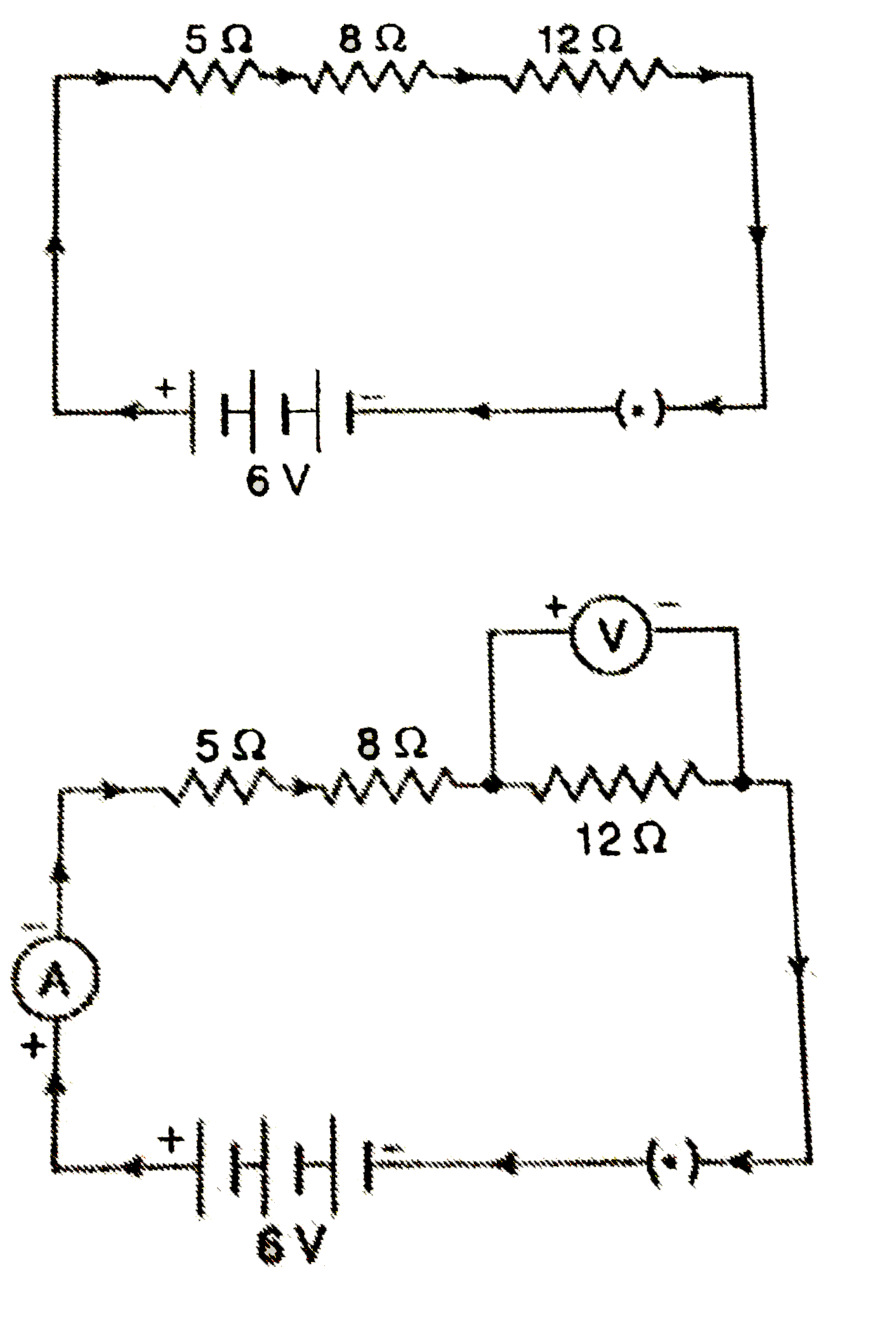# Redraw the circuit of Q.12, putting in an ammeter to measure the current through the resistors and a voltmeter to measure the voltage across the 12

106 views
in Physics
closed
Redraw the circuit of Q.12, putting in an ammeter to measure the current through the resistors and a voltmeter to measure the voltage across the 12 ohm resistor. What would be the readings in the ammeter and the voltmeter ?

by (67.6k points)
selected by

(a) In this problem, we have a battery of 3 cells of 2V each, so the total potential difference (or voltage) of the battery will be 3 xx 2 = 6V. The circuit consisting of a battery of three cells of 2V each (having a voltage of 6V), resistors of 5Omega, 8Omega, 12 Omega and a plug key, all connected in series is given in Figure alongside.
(b) The above circuit can be redraw by including an ammeter in the main circuit and a voltmeter across the 12 Omega resistor, as shown in Figure alongside. Please note that the ammeter has been put in series with the circuit but the voltmeter has been put in parallel with the 12 Omega resistor. We will now calculate the current reading in the ammeter and potential difference reading in the voltmeter.
(i) Calculation of current flowing in the circuit. The three resistors of 5 Omega, 8 Omega and 12 Omega are connected in series. So,
Total resistance, R = 5 +8 +12
= 25 Omega
Potential difference, V = 6V
And, Current, I = ? (To be calculated)
Now, (V)/(I) = R
So, (6)/(I) = 25
25 I = 6
I = (6)/(25)
I = 0.24A
Now, since the current in the circuit is 0.24 amperes, therefore, the ammeter will show a reading of 0.24A.
(ii) Calculation of potential difference across 12 Omega resistor. We have just calculated that a current of 0.24A flows in the circuit. The same current of 0.24A also flows through the 12 Omega resistor which is connected in series. Now, for the 12 Omega resistor:
Current, I = 0.24A (Calculated above)
Resistance, R = 12 Omega (Given)
And, Potential difference, V - ? (To be calculated)
We know that, (V)/(I) = R
So, (V)/(0.24) = 12
And V = 0.24 xx 12
V = 2.88V
Thus, the potential difference across the 12 Omega` resistor is 2.88 volts. So, the voltmeter will show a reading of 2.88 V.